## Review on skin lesion imaging, analysis and automatic classification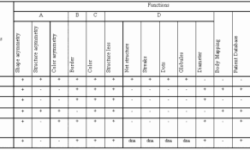The goal of any imaging methodology used in dermatology is to diagnose melanoma in the early stages because it depends on treatment effectiveness. Investigations show that early diagnosis is more than 90% curable and late is less than 50% . The diagnosis and successful treatment are often supplemented with permanent monitoring of suspicious skin lesions. The doctor’s diagnosis is reliable, but this procedure takes lots of time, effort. These routines can be automated. It could save lots of doctor’s time and could help to diagnose more accurately. Besides using computerized means, there is an excellent opportunity to store information with diagnostic information to use it for further investigations or create new methods of diagnosis. Skin lesion imaging methods We found that there are several various imaging methods of skin lesions . The simplest skin visualization method is photography. This method gives only the top layer of the skin image. To get a deeper layer image, there is oil immersion used. It reduces reflections of the surface and brightens the image of the epidermis – the second skin layer.

## Discrete systems in series and parallel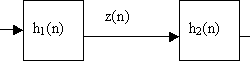Discrete systems in series We have two discrete systems, and their impulse responses are h1(n) and h2(n). Then when these discrete systems are connected in series, then overall impulse response: Where: As you noticed there were changing made: This is nothing more than convolution of impulse responses of both discrete systems: Discrete systems in parallel If we have two discrete systems connected in parallel: As we see, there is a simple sum of output queues of each discrete system. So we can assume, that the overall impulse response is the sum of systems connected in parallel:

## Impulse Response of discrete system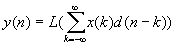Impulse signal can be represented as:d[n] = 1, if n=0d[n] = 0, otherwiseit can also be written like d=[1,0,0,0,â€¦] Impulse Response The impulse response h(n) is the response of filter L() at time n to unit impulse occurring at time 0.h(n)=L(d(n))Let’s see how a discrete system can be described when impulse response is known We know that: In the linear system this can be written as follows: Because h(n-k)=L(d(n-k))Then: What do we get? Its impulse response can describe a linear system. The last expression is called convolution. This is the heart of DSP Filtering.To write this sum in the more convenient matter is assumed that: Matlab example Matlab example: As you can see if the input queue has N samples, impulse response has M after convolution there will be total N+M-1 samples.

## What is a linear system?A discrete system is nothing more than an algorithm, where input is transformed into output. The output is transformed by operator L(), which describes the discrete system.Let’s see the few most common operators of discrete systems. Delay This means that output queue is delayed by on sample. Multiplication This operator takes each sample of input queue and multiplies by constant a. Sum operator Takes two or more sample queues and adds them in the output. Assuming we can say, that the system is linear if input sum reaction is equal to sum of inputs reactions: The system has stable parameters if:y(n-k)=L(x(n-k)), this means that output delay should be the same as the input.It is obvious that delay, multiply, and sum operators are linear and have stable parameters. We will need them for further lessons.

## Understanding of discrete signals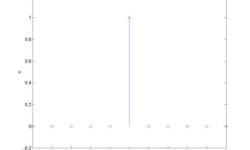Discrete signals can be generated by software or obtained from the real-world through ADC. Discrete signals are sampled from analog signals. So you get samples in fixed time intervals. The discrete signal is a sequence of numbers. The element number n of the sequence is marked as x(n). The most common number rows: Unit sample sequence You can describe it in Matlab like

## Physiological system modeling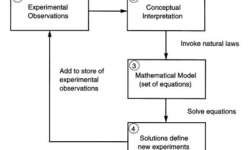Most physical and chemical experiments can be described with a finite number of rules. In physiological processes, everything can be tied to the laws of nature. A good model can be a guideline for new experiments. Usually, the model and experiment have an iterative connection. To understand how to investigate any material, there is a model needed (block 1). First, we need an abstract understanding of what we are investigating (block 2). Abstracts are transformed into mathematical models (block 3). There we can use formulas and solve them using the computer. The results you get shows, what experiments have to be done, and what to expect from them. This loop system is resistive to irregularities in the mathematical model. In other words, this is the purifier of experiments and models.

## CMOS and TTL compliance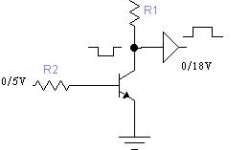There was a time when all IC’s were only TTL type. They were marked as 74LS or 74ALS. But then there came CMOS types of semiconductors. They are usually marked CDxx. And lately, there appeared combined semiconductors that are compliant with both types. They usually are marked as 74HC or 74HCT.TTL is faster than CMOS, but they sink more power. They are powered from a 5V source. To diminish the power consumption, the CMOS was developed. They can work with supplied voltages in the range of 3 to 15V. The main disadvantage is that they are static sensitive. So you always have to be grounded when working with CMOS.Now both types of semiconductors are widely used. Sometimes there is a dilemma to connect TTL to CMOS. You cannot connect TTL directly to CMOS as there are different supply voltages. So it would help if you had some compliance circuit. The simplest can be bipolar transistor cascade. Just remember that such a cascade inverts the signal, so you might need to put an inverter in front of CMOS. Another reminder – never leave TTL or CMOS free pins hanging. Jus connect them through a resistor to VCC or GND.

## Skin lesion boundary tracing algorithm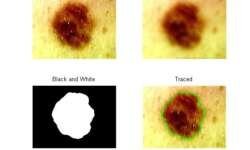I found Matlab to be a convenient tool that easily traces the boundaries of objects in a picture. So I adopted it to skin lesions. This can be used to automatically detect skin irregularities and calculate lesion properties like the asymmetry of shape, or border irregularities, which can help detect melanoma. There are numerous investigations done, so I only put a few examples of how it looks. I will give you my source code so that you can try it on your own. Look at my results: 1) And it also finds the center of mass:

## Skin imaging methods for melanoma diagnosis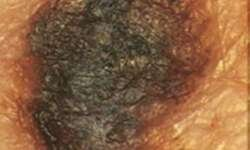There are many skin image capture methodologies developed and used. Here is a short review of them: Dermatoscopic photography The deepest layer of skin can be reached – Papillary dermis Resolution – depends on the optical system View of skin – Horizontal The main disadvantage is reflections of light from skin surface – stratum cornea.

## Polarizer test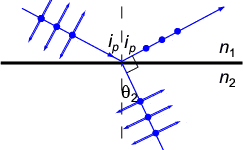I used a simple lamp directed to the glossy table surface. One polarizer is in front of the lamp, and the other is in front of a digital camera lens. Both polarizes perpendicularly oriented to each other. How does this work? A theory about an angle on which the incident polarized electromagnetic waves turn reflects from the surface with a polarization plane turned in 90 degrees. When light going through the polarizer towards the surface, the light is polarized in one direction, and when it reflects from the surface, it is turned by 90 degrees. Another perpendicular polarizer filters those waves in front of the lens.